## WEAKLY COUPLED THERMO-ELECTRIC AND MHD MATHEMATICAL MODELS OF AN ALUMINIUM ELECTROLYSIS CELL

Marc Dupuis* and Valdis Bojarevics**

*GéniSim Inc
3111 Alger St.,Jonquičre, Québec, Canada, G7S 2M9

**University of Greenwich, School of Computing and Mathematics
30 Park Row, London, SE10 9LS, UK
V.Bojarevics@gre.ac.uk

 Abstract In the present study, the full 3D thermo-electric model of a 500 kA demonstration cell has been weakly coupled with the non-linear wave MHD model of the full version of the same cell. In the MHD model, the horizontal ledge distribution calculated in the thermo-electric model has been incorporated as part of the model geometry. In the thermo-electric model, the velocity fields calculated by the MHD model in both the bath and the metal have been used to setup the local heat transfer coefficients at the liquids/ledge interface. Both models have been solved alternatively until a convergence for the horizontal ledge distribution has been obtained. The MHD model has been upgraded with the transiently coupled ferromagnetics solution module, ledge input and with an optimized bus-bar arrangement for a stable 500 kA cell suitable for commercial application. Introduction The mesh of a 3D full cell and external bus-bar thermo-electric model of a 300 kA cell has been presented in 2002 (see figure 10 of ). That 423,296 element model could not be solved on the PIII computer available to the author at the time. More recently, the mesh of a 3D full cell and external bus-bar thermo-electric model of a 500 kA cell has been presented (see figure 6 of ). That 585,016 element model could not be solved either, even on a P4 3.2 GHz computer with 2 GB of RAM. Yet, this is the type of thermo-electric model that the authors want to couple with the non-linear wave MHD model [3, 2] in order to be able to take into consideration the interactions between the thermo-electric and the MHD aspects of the cell behavior . Perseverance finally pays off as these two models could finally be solved. For the first time, the results of those two 3D full cell and external bus-bar thermo-electric models will be presented. 3D full cell and external bus-bar thermo-electric model of the 300 kA demonstration model Since this very first 3D full cell and external bus-bar thermo-electric 423,296 elements model has been built on a PIII computer having only 384 MEG of RAM , it has not even been considered to try to solve it on that hardware. Later on, when the P4 computer with 2GB became available, a bigger 500 kA cell demonstration model was build and the attempt to solve it failed . Taking a step back, it is now possible to attempt to solve the 300 kA cell model on that same P4 computer. It is even possible to take a second step back and try instead to solve a coarser mesh version of that same 300 kA cell model.Figure 1: Coarser mesh of the 3D full cell and external bus-bar thermo-electric 300 kA cell model.

 Figure 1 presents the coarser 249,322 elements mesh that could finally actually be solved. The P4 3.2 GHz computer took 63.7 CPU hours to solve the model once (see the thermal solution in figure 2). Since it took so long to solve, no attempt to converge the ledge profile geometry has been performed.Figure 2: Thermal solution of the 3D full cell and external bus-bar thermo-electric 300 kA cell model. Because of the bus-bar symmetry, one cathode flexible was cut off in order to ensure a non-symmetric solution. That flexible is located near the front side, back side centerline of the cell on the negative or downstream side. Figure 3 presents the resulting metal pad current density.Figure 3: Current density in the metal pad of the 3D full cell and external bus-bar thermo-electric 300 kA cell model. 3D full cell and external bus-bar thermo-electric model of the 500 kA demonstration model The above first successful solution of a 3D full cell and external bus-bar model revealed that the iterative solver, required to solve such big models, is very sensitive to the problem setup. As example, electric boundary conditions can be imposed in many valid ways, but there are ways that will lead to a faster convergence. It was also discovered that the simple fact of cutting one flexible decreased drastically the convergence rate of the iterative solver. This experience was put to use when it was time to try to solve once again the 500 kA demonstration cell model. This time a coarser mesh of 329,288 elements was built (see figure 4) and a more robust set of electric boundary conditions was used. With that setup, it was finally possible to successfully solve the model.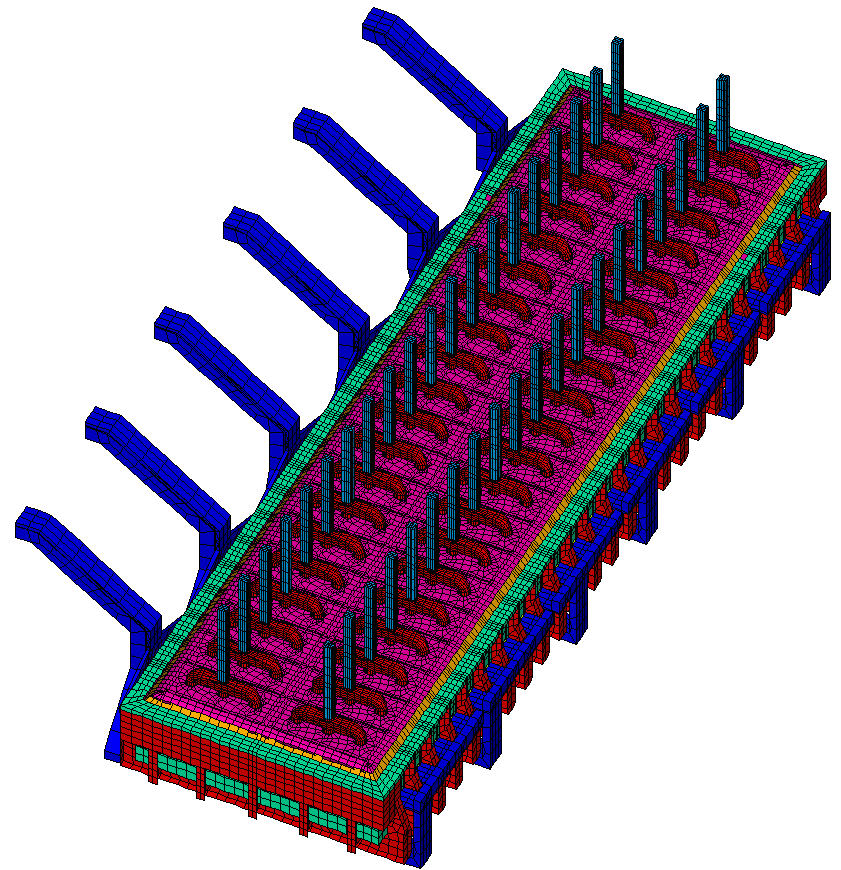Figure 4: . Coarser mesh of the 3D full cell and external bus-bar thermo-electric 500 kA cell model. The P4 computer took "only" 40.6 CPU hours to solve the model 6 times (i.e. the initial solution plus 5 loops of the ledge profile geometry convergence scheme, see figure 5 for the thermal solution). Figure 6 presents the computed current density in the metal pad.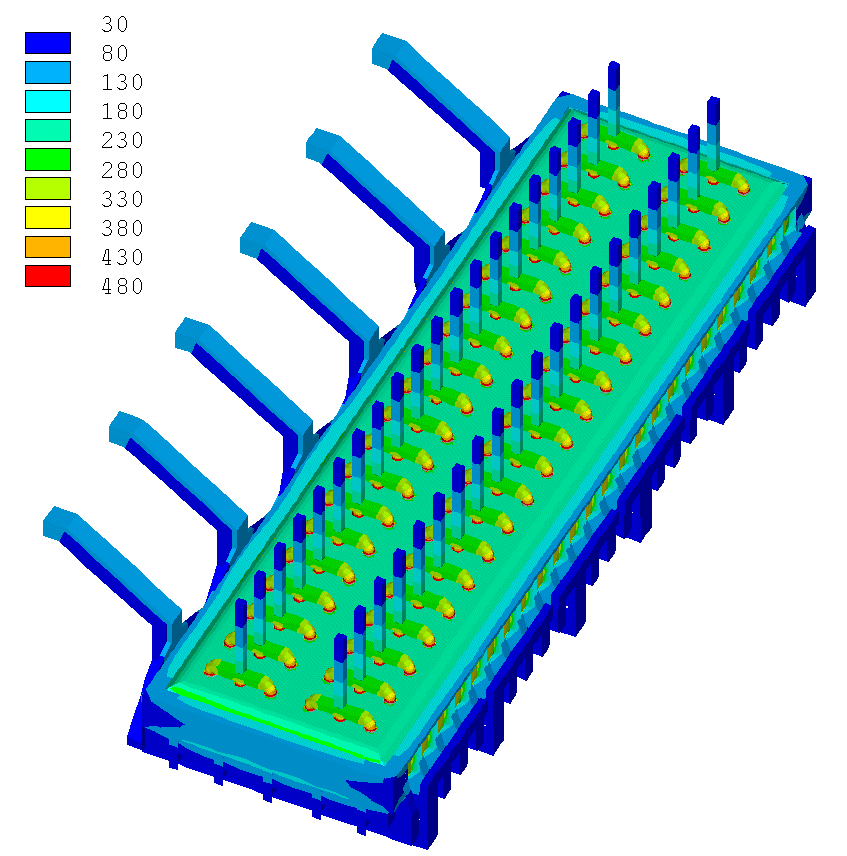Figure 5: Thermal solution of the 3D full cell and external bus-bar thermo-electric 500 kA cell model.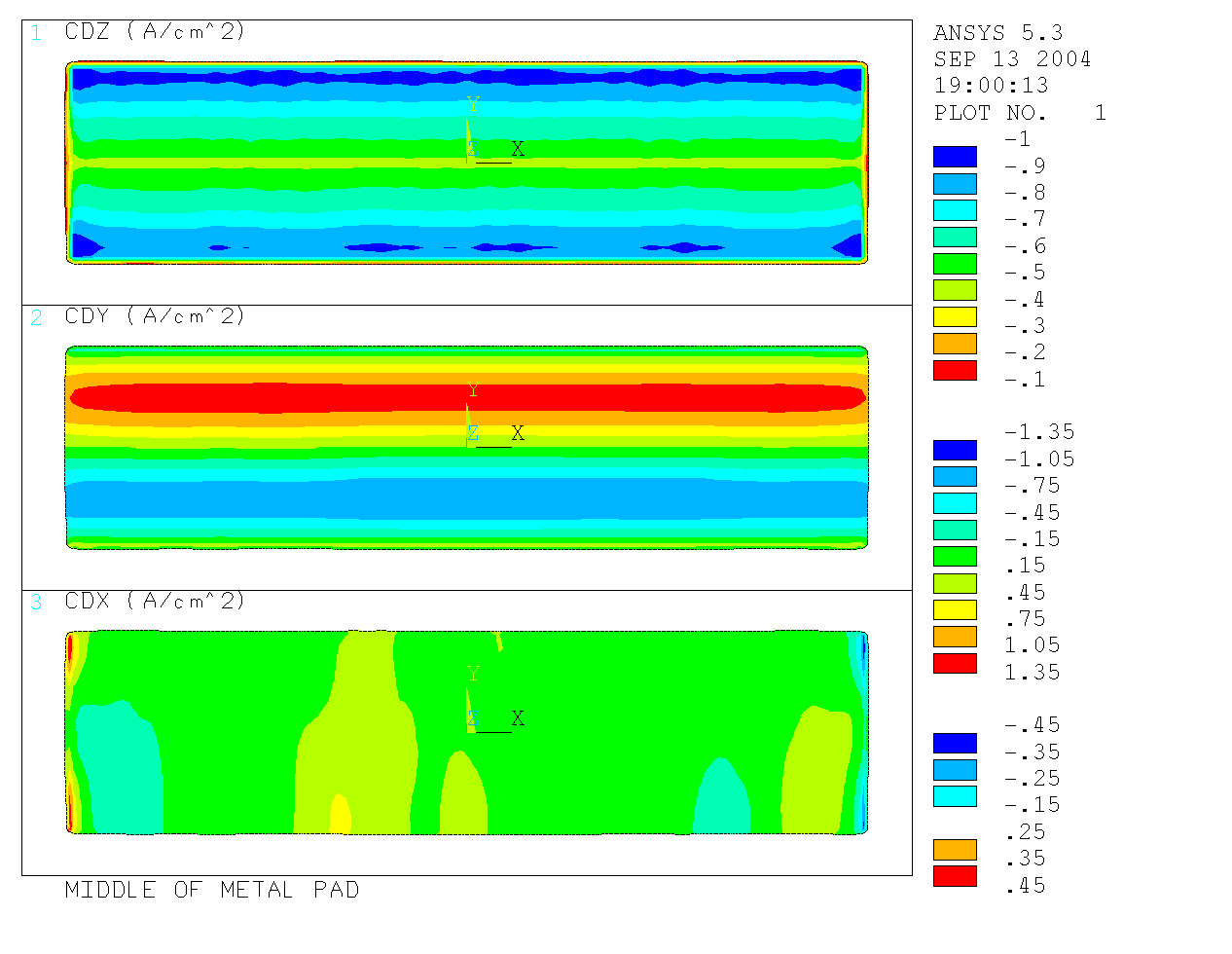Figure 6: Current density in the metal pad of the 3D full cell and external bus-bar thermo-electric 500 kA cell model. MHD model The MHD model of the transient fluid flow coupled to the electromagnetic field at all times [2, 3] has been upgraded to include the ledge toe shape on the bottom input from the thermoelectric model. This means that a significant change in the electric current distribution occurs in the liquid metal, which in turn affects the turbulent velocity field and the cell stability. The cell stability is determined by directly computing the metal-electrolyte interface wave development accounting for the full velocity field and electromagnetic field adjustment at each very small computational time step, typically 0.1-0.25 s for the 500kA cell test runs. A typical run extends to 1000 s of the physical time which is sufficient to achieve a well established wave and flow pattern. An additional upgrade was made to the MHD model by including the ferromagnetics model directly in the full MHD simulation package. There is no need any more to run a separate ferromagnetics program in order to account for the effect of the steel parts magnetization. Instead the present model constantly updates the magnetic field with the magnetization effects by using a fast converging iterative magnetic field solver. This solver has no limit on the ferromagnetic element number dictated by the computer memory restrictions because the influence matrix is not stored. The nested iteration procedure is implemented in such a way that convergence for non-linear material properties and the element mutual interaction is achieved in few iterations. The initial step convergence also is relatively fast (typically in less than 100 iterations). The user of the MHD program package can input the whole steel parts (plates) and the magnetization curve (either measured for the specific materials or taken from the literature). In the present runs we used about 3500 steel elements, and tests with 10 000 elements confirmed no significant change.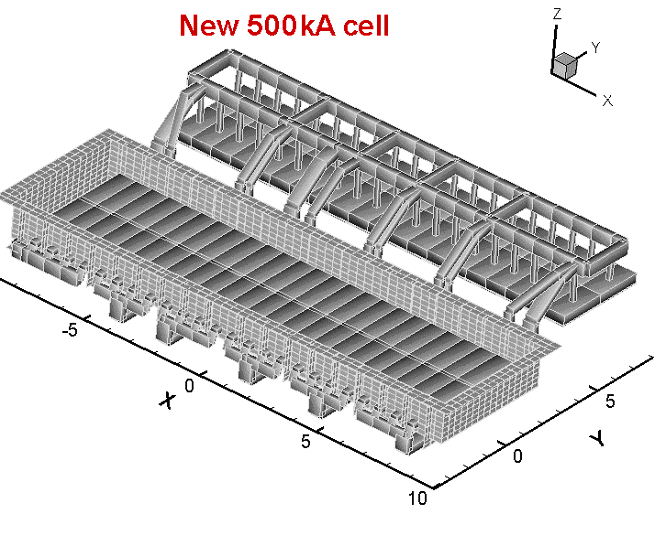Figure 7: Two bus-bar arrangements for the 500 kA cell: 'New' and 'Modified'.

 More work has been done to optimize the bus-bar arrangement for our 'New' 500 kA cell , which actually was only marginally stable and not suitable for practical industrial implementation. The current carrying bus arrangement is shown in Figure 7 together with the steel parts used in the present simulations. The same figure (bottom part) shows the modified bus arrangement which ensures significant reduction of the overall magnetic field, especially the vertical Bz component. Figure 8 demonstrates the comparisons with the previous cell ('New') and the 'Modified' cell total magnetic fields. For such a high amperage cell (500 kA) the magnetic field is well compensated for the 'Modified' cell, the average magnitude of the vertical field being about 0.0012 T. It needs to be mentioned that the parallel row of the cells carrying the return current is positioned very close at 20 m free space between the rows. If this space is increased to 60 m, the vertical field average magnitude is reduced to 0.0008 T which brings the cell in a very favorable MHD regime when even the theoretical stability limits are satisfied.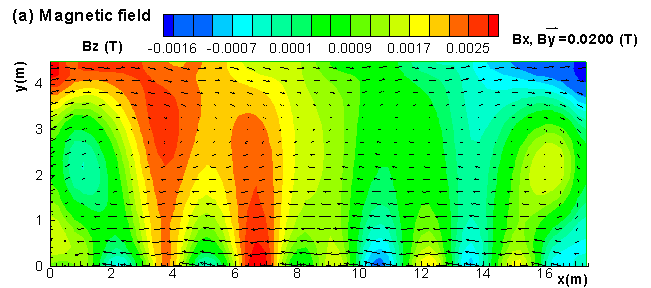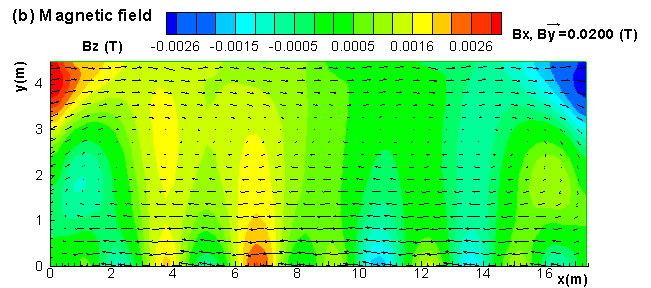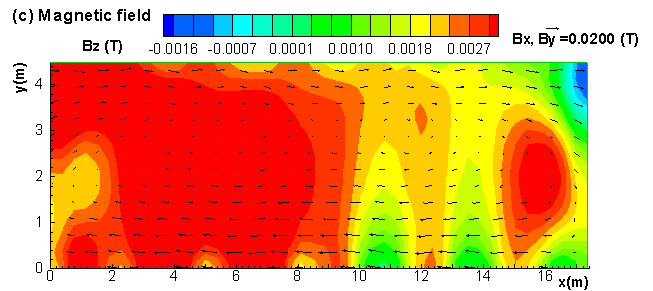Figure 8: Magnetic field distribution for the 500 kA cell: (a) 'Modfied', return row of cells at 20 m (Bmax= 0.0039 T), (b) 'Modified', return row at 60 m (Bmax= 0.0032 T), (c) for previous design cells (Bmax= 0.0053 T). The bus arrangement and their cross-sections were additionally optimized also for the electric current distribution uniformity over the cathode collectors, including the 50:50 split for the upstream and downstream currents. The current distribution in the liquid aluminium is shown in the Figure 9, where the ledge toe profile can be seen as input from the coupled thermoelectric model.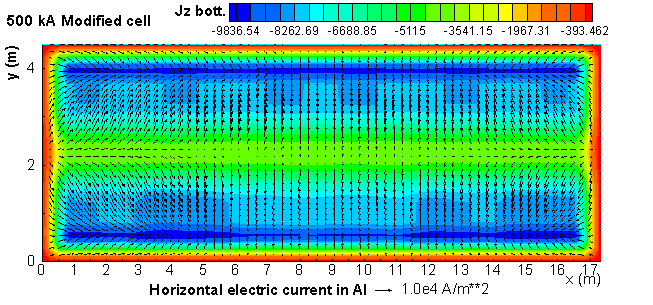Figure 9: Electric current distribution in the liquid metal computed with the MHD model and the ledge position input from the Thermoelectric model: arrows show the horizontal depth averaged current, contour levels - vertical current on the bottom from the top view point. The electromagnetically self-sustained wave and voltage oscillation pattern for the cell is a measure for the cell operation stability. Typically almost all commercial cells even of low amperage 80-100 kA exhibit some rolling wave noise which we can detect also with the present MHD simulation package. Our experience with commercial cell modeling showed the presence of this sustained noise in almost all cell types even at low levels. The present 'Modified' 500kA cell shows relatively low amplitude signal (Figure 10 a,b) at a well defined frequency about 0.017 Hz, which is between 3rd and 4th gravitational self frequencies for this cell. However, a very remarkable result is achieved when moving the return current line to 60 m - the oscillation is completely damped out (Figure 10 c). Therefore we could expect that this cell will be a very good candidate for a commercial implementation, at least from the point of view of the MHD. The initial 'New' cell design was far from this very stable condition, as can be seen from Figure 10 d. Note, also the predicted voltage oscillation detectable from this model, and which can not be detected by the many other MHD models. A 'stationary' interface shape in practice is never achieved, because the real cells operate in transient regimes. Even the MHD rolling wave noise is quite typical for many cells. The 'New' 500 kA cell shows quite considerable wave pattern and the Figure 11a presents the interface shape at a particular time moment 500 s from the simulation start. Clearly the 'Modified' version of the cell has much better interface shape of lower amplitude (Figure 11b). Here the small humps and depressions are created by the pressure differences in the two layers owing to the difference in the turbulent large scale recirculation patterns. When the return current line was moved to 60 m distance, the interface can achieve a shape which is practically stationary and of very little deformation as shown in the Figure 11c. The horizontal velocity field is not damped, as shown in Figure 12, even for the stable 'Modified' cell. However, the turbulent average velocity pattern is relatively symmetric and it is very similar both in the liquid metal and the electrolyte, therefore the horizontal flow generated pressure distribution is also well compensated at the interface and no significant additional deformation is created for the stable cell.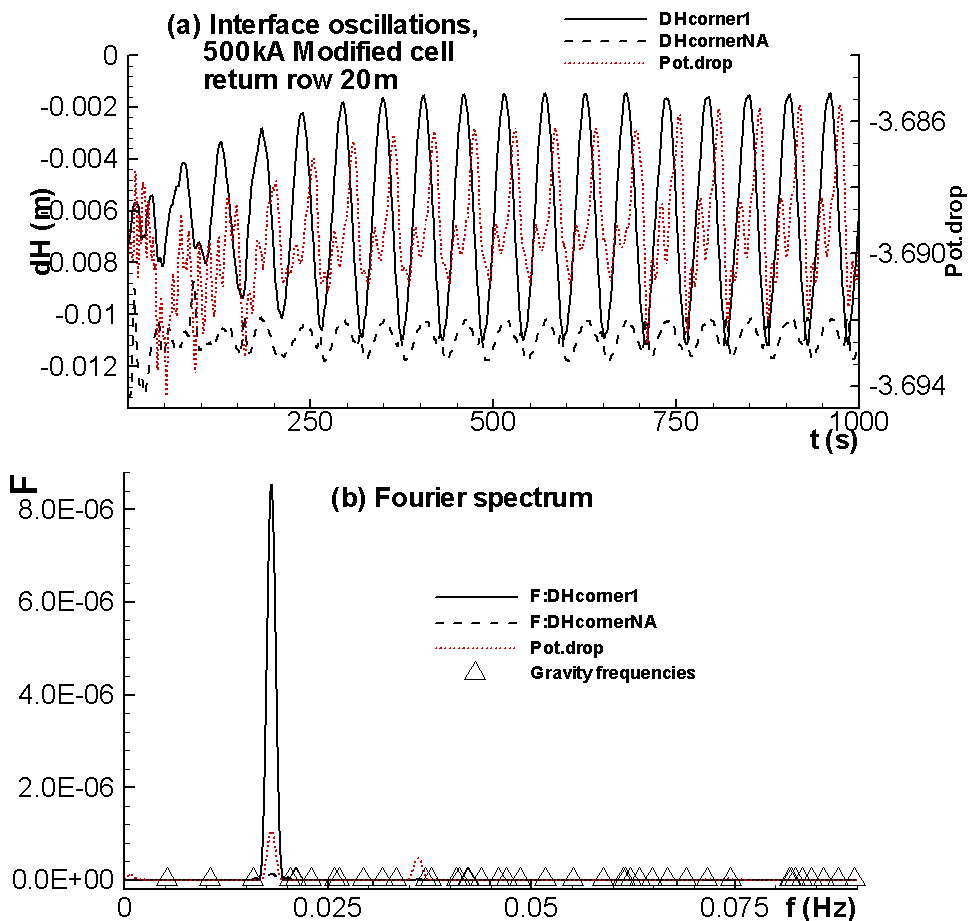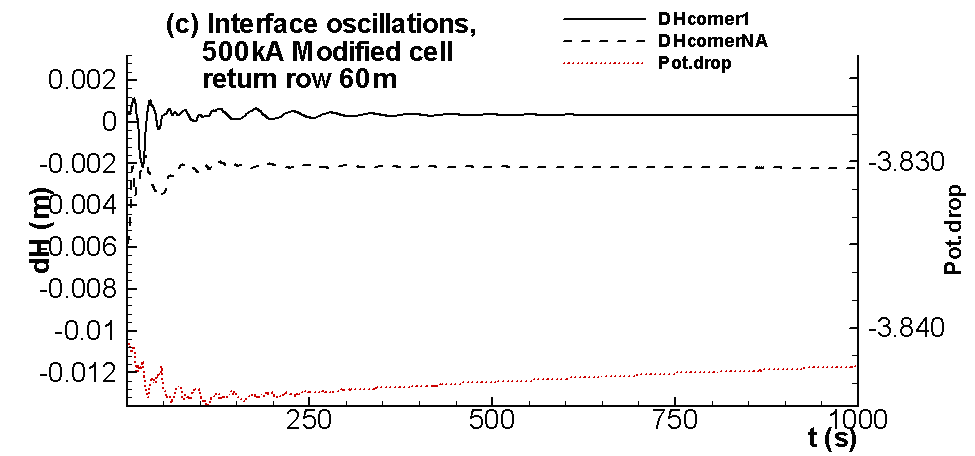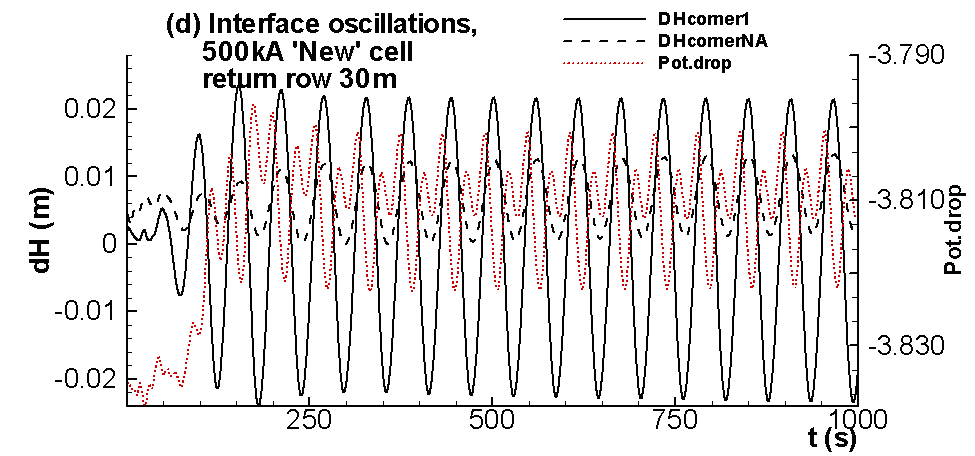Figure 10: Metal-bath interface oscillations at two diagonally opposite corners (under anode N1 and anode N40) and the voltage oscillation: (a) for modified cell, return row of cells at 20 m, (b) Fourier spectra for the case a), (c) for modified cell, return row at 60 m, (d) for previous design cells. The velocity pattern and magnitude is still important for the additive transport (alumina) and for the temperature distribution highly affected by the turbulent effective heat transfer. The turbulent effective transport coefficients are similar for electrolyte and the liquid metal layers, which makes them both much better thermal transport media if compared to the quiescent or laminar conditions. Therefore special heat transfer and ledge formation characteristics need to be considered usually based on semi-empirical models. One possible approach to this problem is considered in the next section.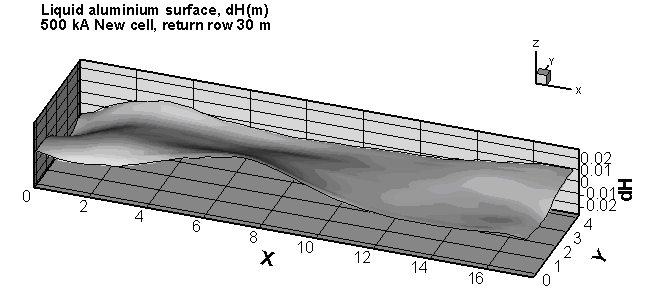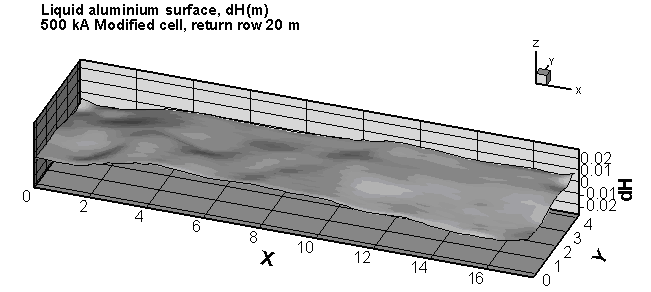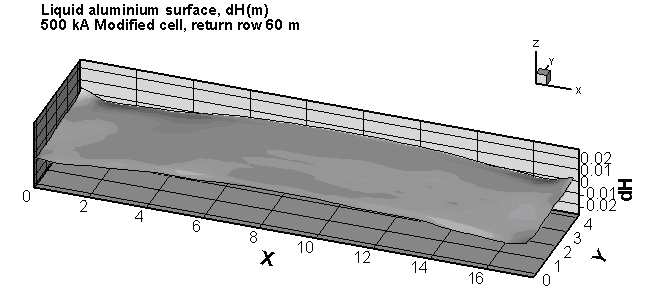Figure 11: Interface shapes of liquid aluminium for the 500 kA cell at approximately t=500 s: (a) 'New', (b) and (c) 'Modified' (return line at 20 and 60 m respectively).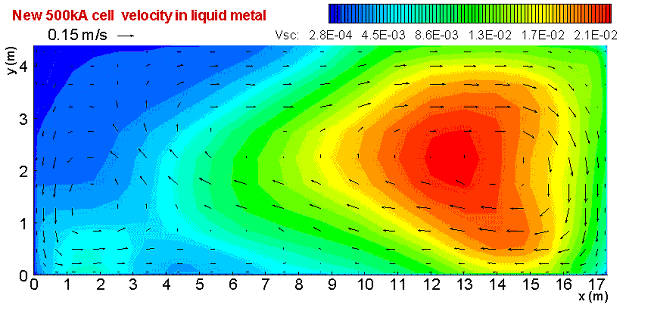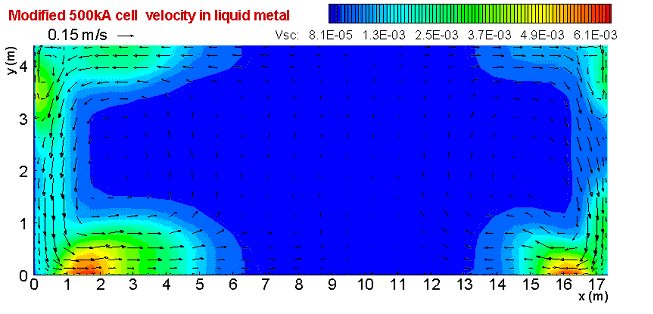Figure 12: Velocity fields and turbulent effective viscosity distribution in liquid aluminium for the 500 kA cell: 'New' and 'Modified'.Weakly coupled thermo-electric and MHD run

As described in , the velocity dependent local heat transfer coefficients at the bath/ledge and the metal/ledge interfaces are at the heart of the coupling between the thermo-electric model and the MHD model.

Many relationships between the bath velocity and the local heat transfer coefficient at the bath/ledge interface have been proposed in the literature, some of them have been presented in . In the first coupling trial presented in , the following relationships were selected: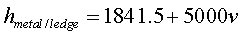(1)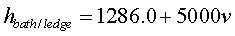(2)

Where h is in W/m˛ °C and v is in m/s. As in the MHD model the velocity (v) is set to zero at the ledge surface, the velocity used in Equations (1) and (2) is the bulk fluid velocity in the immediate vicinity of the ledge surface.

Those direct linear relationships mostly inspired by Nazeri  did not produce very substantially varying local values for the heat transfer coefficients along the cell perimeter. This in turn leads to the calculation of a fairly uniform ledge toe position along the cell perimeter in the thermo-electric model. Of course, in the complete absence of experimental data, it is very hard to assess the accuracy of that prediction.

Yet, since the principal goal of the present work is to develop the convergence strategy of the weakly coupled thermo-electric and MHD models, it is important to get a significant coupling feedback loop between the two models. For that reason, a more intense and non-linear relationships for small velocity regime mostly inspired by Fletcher  were used in the present work: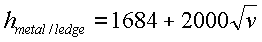(3)(4)

As in the previous work , the coefficients 1684 and 1121 were selected in order to get average values identical to the constant values used before, as typical values of the heat transfer coefficients are estimated to be around 2000 W/m2K when using the cell liquidus superheat. The choice of the coefficient of 2000 (W/m2K)/(m/s)˝ is extracted directly from Fletcher equation (eq. 3 in ).

This very first weakly coupled thermo-electric and MHD run was carried out as follow:

 1 Solution of the thermo-electric full cell model using constant heat transfer coefficients of 1425 W/m2K at the bath/ledge interface and 2052 W/m2K at the metal/ledge interface. Notice that this cell operates in thermal balance at 9.3 şC of liquidus superheat. In principle, the ledge profile geometry convergence loop must be carried out until all the nodes on the liquids/ledge interfaces reach the liquidus temperature of 953.7 şC. In practice, only 5 iterations were carried out in order to limit the required CPU time to 41 hours. 2 Solution of the MHD model using the ledge toe profile obtained from the above thermo-electric run. The full 1000
 seconds fully non-linear analysis using 0.5 seconds time steps requires about 8 CPU hours on the same P4 3.2 GHz computer. 3. Solution of the thermo-electric full cell model using local heat transfer coefficients computed using the MHD model computed flow field and equations (3) and (4) (see figure 13). Again, in order to limit the required CPU time, only 5 ledge profile geometry convergence iterations were carried out. The resulting ledge profile is presented in figure 14. Notice that the cell remained in thermal balance at the same superheat because the constant terms of equations (3) and (4) were selected to ensure that the average heat transfer coefficients remained the same. 4. 4) Solution of the MHD model using the ledge toe profile computed in step 3. Since the new ledge toe profile is only marginally different from the initial one, the flow field computed in step 4 is almost identical to the one computed in step 2. For that reason, it was decided to stop the weakly coupled convergence loop at that point.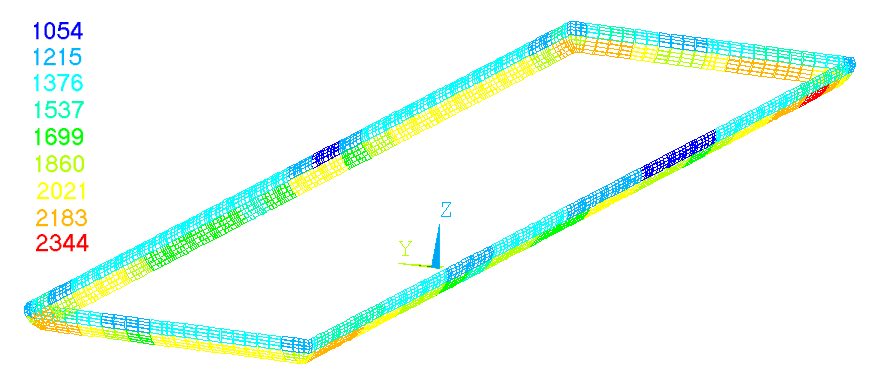Figure 13: Local heat transfer coefficients boundary conditions used in step 3.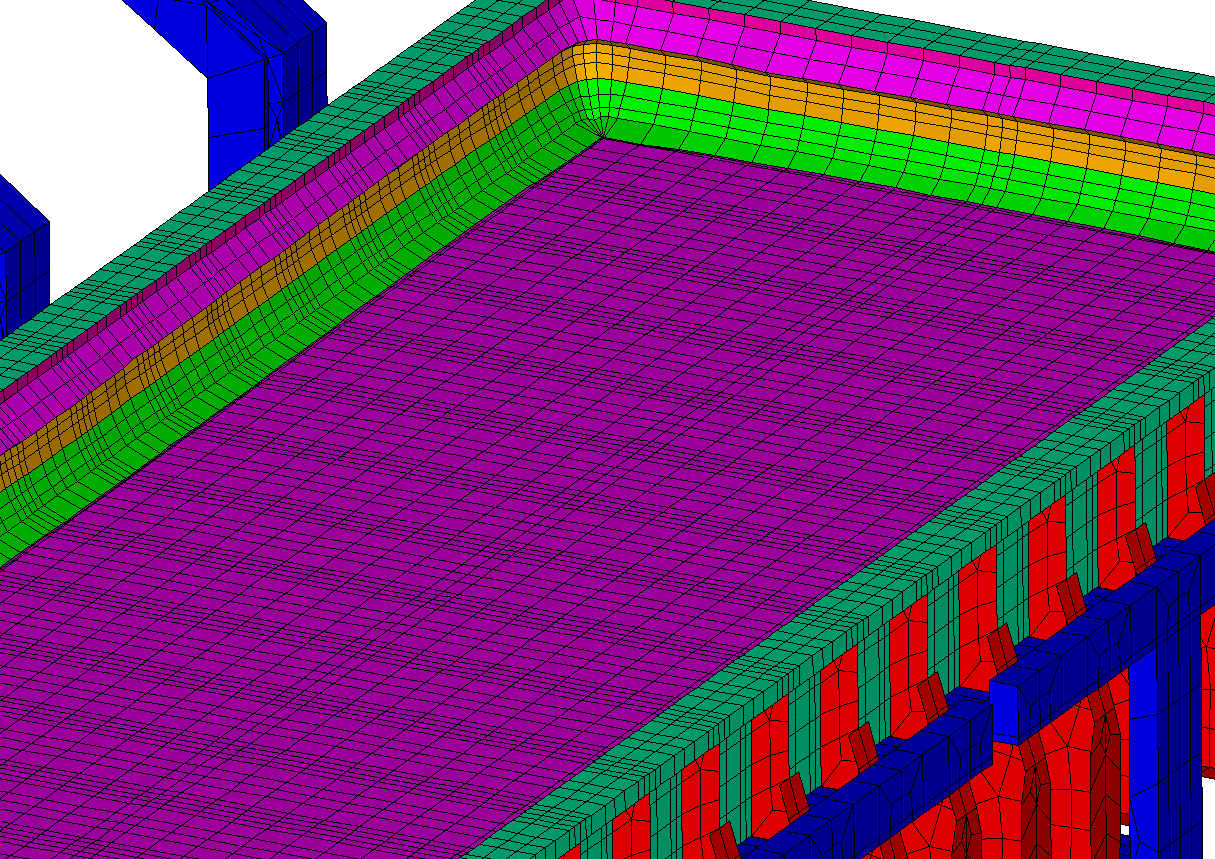Figure 14: Corresponding ledge profile computed in step 3.

Conclusions

A 3D full cell and external bus-bar thermo-electric model was developed and successfully solved. The initial 5 loops ledge profile convergence calculations took 41 CPU hours on a P4 3.2 GHz computer.

 The MHD model has been upgraded with the transiently coupled ferromagnetics solution module, ledge input and with an optimized bus-bar arrangement for a stable 500 kA cell suitable for commercial application. A first weakly coupled thermo-electric and MHD run has been successfully carried out. Only two iterations of the weakly coupled convergence loop were required because the non-linear coupling effects turned out to be not very significant in the present case. Nevertheless, those two iterations required 98 CPU hours on a P4 3.2 GHz computer. References M. Dupuis, "Toward the Development of a 3D Full Cell and External Busbars Thermo-Electric Model", Proceedings of the 41st Conference on Light Metal, CIM, (2002), 25-39. M. Dupuis, V. Bojarevics and J. Freibergs, "Demonstration Thermo-Electric and MHD Mathematical Models of a 500 kA Al Electrolysis cell: Part 2", Light Metals, TMS, (2004), 453-459. M. Dupuis, V. Bojarevics and J. Freibergs, "Demonstration Thermo-Electric and MHD Mathematical Models of a 500 kA Al Electrolysis cell", Proceedings of the 42nd Conference on Light Metals, CIM, (2003), 3-20. M. Dupuis, "Using ANSYS to model aluminum reduction cell since 1984 and beyond", Proceedings of the ANSYS 10th International Conference, (2002), electronic format only. H. Nazeri, T.A. Utigard and P. Desclaux, "The Measurement of Heat Transfer Coefficient between Cryolite and Ledge", CIM Light Metals, (1994), 543-559. FLETCHER, T.E., "The Role of Convective Heat Transfer in Sidewall Ledging", Master thesis, University of Toronto, Canada, (1995).